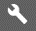## Excel 2013

1. To change column A to Title Case, select cell B2. Type =PROPER(A2), and press Enter. Tip: Use the formula =UPPER(A1) for all UPPERCASE; =LOWER(A1) for all lowercase.

2. Now fill down the formula through cell B10.## Excel 2016

1. To change column A to Title Case, select cell B2. Type =PROPER(A2), and press Enter. Tip: Use the formula =UPPER(A1) for all UPPERCASE; =LOWER(A1) for all lowercase.

2. Now fill down the formula through cell B10.